# Star Delta Starter

The Star Delta Starter is a very common type of starter and is used extensively as compared to the other type of starting methods of the induction motor. A star delta is used for a cage motor designed to run normally on the delta-connected stator winding. The connection of a three-phase induction motor with a star-delta starter is shown in the figure below:When switch S is in the START position, the stator windings are connected in the star as shown below: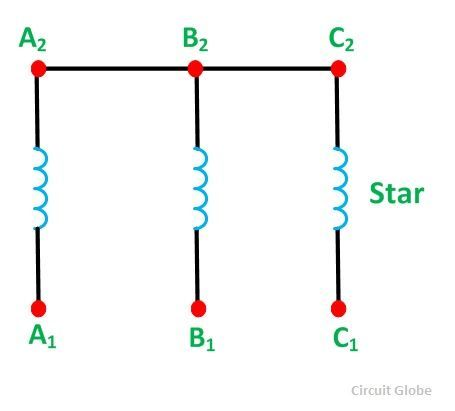When the motor picks up the speed, about 80 percent of its rated speed, the switch S is immediately put into the RUN position. As a result, a stator winding which was in star connection is changed into DELTA connection now. The delta connection of the stator winding is shown in the figure below: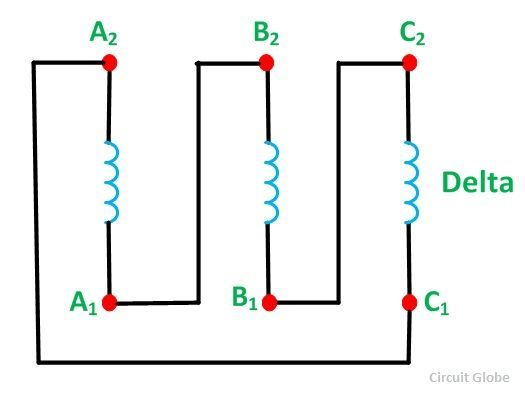Firstly, the stator winding is connected in star and then in Delta so that the starting line current of the motor is reduced to one-third as compared to the starting current with the windings connected in delta. At the starting of an induction motor when the windings of the stator are star-connected, each stator phase gets a voltage VL/√3. Here VL is the line voltage.

Since the developed torque is proportional to the square of the voltage applied to an induction motor. Star delta starter reduces the starting torque to one-third that is obtained by direct delta starting.

## Theory of Star Delta Starter with method of starting of Induction Motor

At the starting of the induction motor, stator windings are connected in star and, therefore, the voltage across each phase winding is equal to 1/√3 times the line voltage.

Let,

• VL is the line voltage,
• Istyp is the starting current per phase with the stator windings connected in star,
• Istyl is the starting line current with the stator winding in the star.

For star connection, the line current is equal to the phase current. Therefore,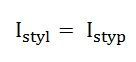If,

• V1 is the phase voltage,
• VL is the line voltage,
• IstΔp is the starting current per phase by direct switching with the stator windings connected in delta,
• IstΔl is the starting line current by direct switching with the stator windings in delta,
• IscΔp is the short circuit phase current by direct switching with the stator windings in delta,
• Ze10 is the standstill equivalent impedance per phase of the motor, referred to as stator.For Delta connection, the line current is equal to the root three times the phase current.

Therefore,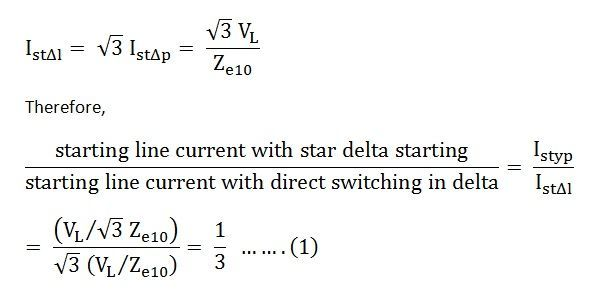Thus, with star delta starter, the starting current from the main supply is one-third to that of direct switching in the delta.

Also,Hence, with star delta starting, the starting torque is reduced to one-third of the starting torque obtained with the direct switching in the delta.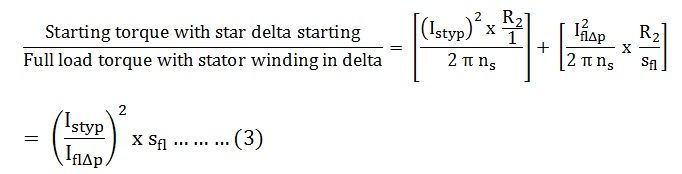Where,

IflΔp is the full load phase current with the winding in the Delta

But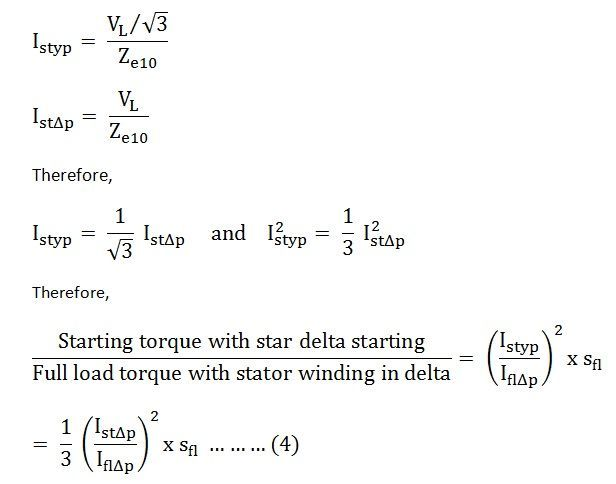Hence, equation (4) shown above gives the starting torque of an induction motor in the star delta starting method.

### 26 thoughts on “Star Delta Starter”

1. Thank you so much
Excellent information in a simple language
It is very helpful to me…..
Once again thank you…….

2. Star delta starters reduces the starting torque to 1/3, and that can be achived by direct delta running is it correct.

3. Sir in star delta starter timer sec is 10 sec . But the contactor changing star to delta immediately why?

4. Bishnu Charan Mahanta

Really easy to understand about starting of induction motor by star-delta starter, thank you.